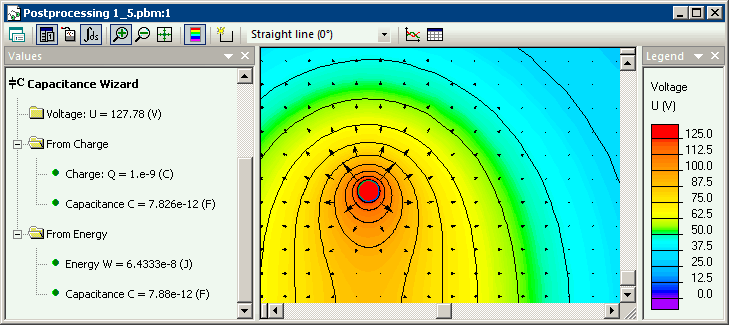QuickField

A new approach to field modelling
 Language: Global English Deutch Espanol Francais Italiano Danmark Ceske Chinese Pycckuü

Main >> Applications >> Sample problems >> Wire ring capacitance

# Wire ring capacitance

Problem Type:

Axisymmetric problem of electrostatics.

Geometry:

R = 100 mm, a = 8.74 mm.

Given:

Relative permittivity of vacuum ε = 1,
The charge q = 10-9 C

Find the wire ring capacitance and compare it with analytical solution:
C = 4πεε0 · πR / ln(8R/a), [F]. *

Solution:

The capacitance can be calculated as C = q /U, where U is a body potential and q is a body charge. Conductor's surface is marked as 'floating conductor', i.e. isolated conductor with unknown potential. At some point on conductor's surface the charge is applied. The charge is then redistributed along the conductor surface automatically.
It is supposed that field fades to zero far away from the ring. The calculation area is limited by the outer boundary, where zero potential is applied.

Results:

Potential distribution.As the potential of the body is calculated with respect to the zero potential of the 'infinity', the result depends on the distance to the outer boundary. The set of simulations where made using automation tool LabelMover.
 Distance to the outer boundary Voltage, V Capacitance, pF 10*R 119.2 8.39 20*R 123.7 8.08 40*R 125.9 7.94 80*R 127.0 7.87 160*R 127.6 7.84 320*R 127.8 7.82 Theory 7.74

• View simulation report in PDF.

• Download simulation files (files may be viewed using any QuickField Edition).

*"The Capacitance of an Anchor Ring" by Thomas, T. S. E, Australian Journal of Physics, vol. 7, p.347.